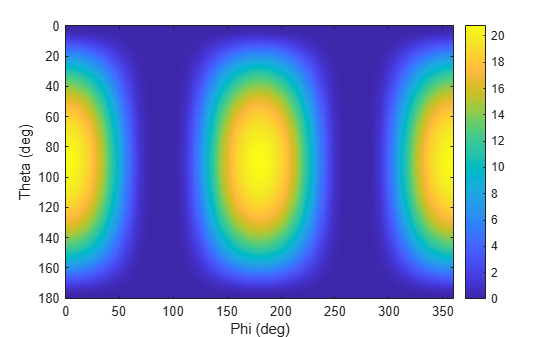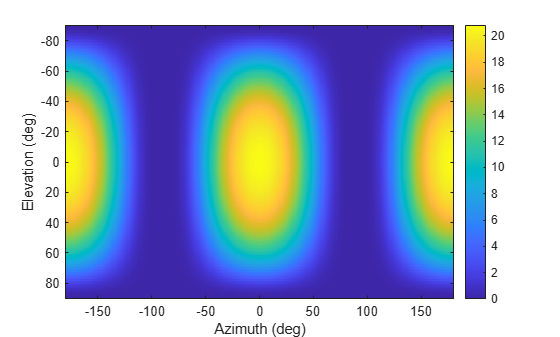# phitheta2azelpat

Convert radiation pattern from phi-theta coordinates to azimuth-elevation coordinates

## Syntax

``pat_azel = phitheta2azelpat(pat_phitheta,phi,theta)``
``pat_azel = phitheta2azelpat(pat_phitheta,phi,theta,az,el)``
``pat_azel = phitheta2azelpat(___,'RotateZ2X',rotpatax)``
``````[pat_azel,az_pat,el_pat] = phitheta2azelpat(___)``````

## Description

example

````pat_azel = phitheta2azelpat(pat_phitheta,phi,theta)` converts the antenna radiation pattern, `pat_phitheta`, from phi and theta coordinates to the pattern `pat_azel` in azimuth and elevation coordinates. `phi` and `theta` are the phi and theta coordinates at which `pat_phitheta` values are defined. The `pat_azel` matrix covers azimuth values from –180 to 180 degrees and elevation values from –90 to 90 degrees in one degree increments. The function interpolates the `pat_phitheta` matrix to estimate the response of the antenna in a given direction.```

example

````pat_azel = phitheta2azelpat(pat_phitheta,phi,theta,az,el)` uses vectors `az` and `el` to specify the grid at which to sample `pat_azel`. To avoid interpolation errors, `az` should cover the range [–180, 180] and `el` should cover the range [–90, 90].```

example

````pat_azel = phitheta2azelpat(___,'RotateZ2X',rotpatax)` also specifies `rotpatax` to indicate the boresight direction of the pattern: x-axis or z-axis.```

example

``````[pat_azel,az_pat,el_pat] = phitheta2azelpat(___)``` also returns vectors `az_pat` and `el_pat` containing the azimuth and elevation angles at which `pat_azel` is sampled.```

## Examples

collapse all

Convert a radiation pattern to azimuth/elevation form, with the azimuth and elevation angles spaced 1° apart.

Define the pattern in terms of φ and θ.

```phi = 0:360; theta = 0:180; pat_phitheta = mag2db(repmat(cosd(theta)',1,numel(phi)));```

Convert the pattern to azimuth/elevation space.

`pat_azel = phitheta2azelpat(pat_phitheta,phi,theta);`

Convert a radiation pattern from theta/phi coordinates to azimuth/elevation coordinates, with azimuth and elevation angles spaced ${1}^{\circ }$ apart.

Define the pattern in terms of phi, $\varphi$, and theta, $\theta$, coordinates.

```phi = 0:360; theta = 0:180; pat_phitheta = mag2db(repmat(cosd(theta)',1,numel(phi)));```

Convert the pattern to azimuth/elevation coordinates. Get the azimuth and elevation angles for use in plotting.

`[pat_azel,az,el] = phitheta2azelpat(pat_phitheta,phi,theta);`

```H = surf(az,el,pat_azel); H.LineStyle = 'none'; xlabel('Azimuth (degrees)'); ylabel('Elevation (degrees)'); zlabel('Pattern');```Convert a radiation pattern to the azimuth-elevation coordinates from alternative phi-theta coordinates, with the phi and theta angles spaced one degree apart.

Create a simple radiation pattern in terms of phi and theta. Add an offset to the pattern to suppress taking the logarithm of zero in mag2db.

```phi = 0:360; theta = 0:180; pat_phitheta = mag2db(10*sind(theta').^2*cosd(phi).^4 + 1); imagesc(phi,theta,pat_phitheta) xlabel('Phi (deg)') ylabel('Theta (deg)') colorbar``````[pat_azel,az_pat,el_pat] = phitheta2azelpat(pat_phitheta,phi,theta,'RotateZ2X',false); imagesc(az_pat,el_pat,pat_azel) xlabel('Azimuth (deg)') ylabel('Elevation (deg)') colorbar```Convert a radiation pattern from phi/theta coordinates to azimuth/elevation coordinates, with the azimuth and elevation angles spaced ${5}^{\circ }$ apart.

Define the pattern in terms of phi and theta.

```phi = 0:360; theta = 0:180; pat_phitheta = mag2db(repmat(cosd(theta)',1,numel(phi)));```

Define the set of azimuth and elevation angles at which to sample the pattern. Then, convert the pattern.

```az = -180:5:180; el = -90:5:90; pat_azel = phitheta2azelpat(pat_phitheta,phi,theta,az,el);```

```H = surf(az,el,pat_azel); H.LineStyle = 'none'; xlabel('Azimuth (degrees)'); ylabel('Elevation (degrees)'); zlabel('Pattern');```## Input Arguments

collapse all

Antenna radiation pattern in phi-theta coordinates, specified as a real-valued Q-by-P matrix. `pat_phitheta` contains the magnitude pattern. P is the length of the `phi` vector, and Q is the length of the `theta` vector. Units are in dB.

Data Types: `double`

Phi angles at which `pat_phitheta` is sampled, specified as a vector of real-valued length-P vector. Phi angles lie between 0 and 360, inclusive. Units are in degrees.

Data Types: `double`

Theta angles at which `pat_phitheta` is sampled, specified as a vector of real-valued length-Q vector. Theta angles lie between 0 and 180, inclusive. Units are in degrees.

Data Types: `double`

Azimuth angles at which `pat_azel` samples the pattern, specified as a vector of real-valued length-L vector. Azimuth angles lie between –180 and 180, inclusive. Units are in degrees.

Data Types: `double`

Elevation angles at which `pat_azel` samples the pattern, specified as a real-valued length-M vector. Elevation angle lie between –90 and 90, inclusive. Units are in degrees.

Data Types: `double`

Pattern boresight direction selector, specified as `true` or `false`.

• If `rotpatax` is `true`, the pattern boresight is along the x-axis. In this case, the z-axis of phi-theta space is aligned with the x-axis of azimuth and elevation space. The phi angle is defined from the y-axis to the z-axis and the theta angle is defined from the x-axis toward the yz-plane. (See Phi and Theta Angles).

• If `rotpatax` is `false`, the phi angle is defined from the x-axis to the y-axis and the theta angle is defined from the z-axis toward the xy-plane. (See Alternative Definition of Phi and Theta).

Data Types: `logical`

## Output Arguments

collapse all

Antenna radiation pattern in azimuth-elevation coordinates, returned as a real-valued M-by-L matrix. `pat_azel` represents the magnitude pattern. L is the length of the `az_pat` vector, and M is the length of the `el_pat` vector. Units are in dB.

Azimuth angles at which the `pat_azel` output pattern is sampled, returned as a real-valued length-L vector. Units are in degrees.

Elevation angles at which the `pat_azel` output pattern is sampled, returned as a real-valued length-M vector. Units are in degrees.

collapse all

### Azimuth and Elevation Angles

The azimuth angle of a vector is the angle between the x-axis and the orthogonal projection of the vector onto the xy plane. The angle is positive in going from the x axis toward the y axis. Azimuth angles lie between –180 and 180 degrees. The elevation angle is the angle between the vector and its orthogonal projection onto the xy-plane. The angle is positive when going toward the positive z-axis from the xy plane. By default, the boresight direction of an element or array is aligned with the positive x-axis. The boresight direction is the direction of the main lobe of an element or array.

Note

The elevation angle is sometimes defined in the literature as the angle a vector makes with the positive z-axis. The MATLAB® and Phased Array System Toolbox™ products do not use this definition.

This figure illustrates the azimuth angle and elevation angle for a vector shown as a green solid line.### Phi and Theta Angles

The phi angle (φ) is the angle from the positive y-axis to the vector’s orthogonal projection onto the yz plane. The angle is positive toward the positive z-axis. The phi angle is between 0 and 360 degrees. The theta angle (θ) is the angle from the x-axis to the vector itself. The angle is positive toward the yz plane. The theta angle is between 0 and 180 degrees.

The figure illustrates phi and theta for a vector that appears as a green solid line.The coordinate transformations between φ/θ and az/el are described by the following equations

`$\begin{array}{l}\mathrm{sin}el=\mathrm{sin}\varphi \mathrm{sin}\theta \\ \mathrm{tan}az=\mathrm{cos}\varphi \mathrm{tan}\theta \\ \mathrm{cos}\theta =\mathrm{cos}el\mathrm{cos}az\\ \mathrm{tan}\varphi =\mathrm{tan}el/\mathrm{sin}az\end{array}$`

### Alternative Definition of Phi and Theta

The phi angle (φ) is the angle from the positive x-axis to the vector’s orthogonal projection onto the xy plane. The angle is positive toward the positive y-axis. The phi angle is between 0 and 360 degrees. The theta angle (θ) is the angle from the z-axis to the vector itself. The angle is positive toward the xy plane. The theta angle is between 0 and 180 degrees.

The figure illustrates φ and θ for a vector that appears as a green solid line.`$\begin{array}{l}\varphi =az\\ \theta =90-el\\ az=\varphi \\ el=90-\theta \end{array}$`